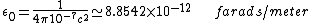# permittivity

Also found in: Dictionary, Wikipedia.
Related to permittivity: dielectric constant

## Permittivity

A property of a dielectric medium that determines the forces that electric charges placed in the medium exert on each other. If two charges of q1 and q2 coulombs in free space are separated by a distance r meters, the electrostatic force F newtons acting upon each of them is proportional to the product of the charges and inversely proportional to the square of the distance between them. Thus, F is given by Eq. (1),

(1)where 1/(4&pgr;ε0) is the constant of proportionality, having the magnitude and dimensions necessary to satisfy Eq. (1). This condition leads to a value for ε0, termed the permittivity of free space, given by Eq. (2), where
(2)c is the velocity of light in vacuum.

If now the charges are placed in a dielectric medium that is homogeneous and isotropic, the force on each of them is reduced by a factor εr, where εr is greater than 1. This dimensionless scalar quantity is termed the relative permittivity of the medium, and the product ε0εr is termed the absolute permittivity ε of the medium.

A consequence is that if two equal charges of opposite sign are placed on two separate conductors, then the potential difference between the conductors will be reduced by a factor εr when the conductors are immersed in a dielectric medium compared to the potential difference when they are in vacuum. Hence a capacitor filled with a dielectric material has a capacitance εr times greater than a capacitor with the same electrodes in vacuum would have. Except for exceedingly high applied fields, unlikely normally to be reached, εr is independent of the magnitude of the applied electric field for all dielectric materials used in practice, excluding ferroelectrics. See Capacitance, Capacitor, Ferroelectrics

## permittivity

[‚pər·mə′tiv·əd·ē]
(electricity)
The dielectric constant multiplied by the permittivity of empty space, where the permittivity of empty space (ε0) is a constant appearing in Coulomb's law, having the value of 1 in centimeter-gram-second electrostatic units, and of 8.854 × 10-12 farad/meter in rationalized meter-kilogram-second units. Symbolized ε.
References in periodicals archive ?
Figure 1 (a, b, and c) shows the temperature/pressure dependence of dielectric permittivity [epsilon](T) (at heating).
ii) The characteristics of current pulse generated and the UHF signal formed during surface discharge process, (iii) To analyze permittivity and tan([delta]) variation of epoxy silicon carbide nanocomposite at different frequencies and at different temperatures (iv) To characterize the damage caused due to surface discharge through surface charge measurement and by surface roughness measurement studies, (v) Phase resolved partial discharge (PRPD) studies to identify the phase window of surface discharge occurrences and at higher voltages.
We calculated the end of acceleration time (EAT) as an index of coagulability from temporal changes in dielectric permittivity," coauthor Satomi Hamada says.
The PDIV of PEEK resin decreases at high temperature and this is thought to be due to an increase in permittivity at the glass transition point (150[degrees]C) or higher temperatures.
2] are the permittivity of dielectric and metal layers that are combined to form AMM respectively and N is the volume fraction of the first medium.
The metalomagnetic isotropic inclusions properties of the photonic crystal will be fully described by two material parameters: the permittivity and permeability, which are position functions; therefore,
Considering these premises and the requirement to model a more general permittivity function, in this paper we illustrate a more accurate study of the time-domain electromagnetic field propagation in arbitrary dispersive media.
Extraction of complex permittivity using the above technique requires physical assumptions be made regarding the emitted electromagnetic wave from the antenna and its interaction with the test sample.
Among specific topics are the complex permittivity of soils in western Rajasthan at microwave frequency, Compton scattering and electronic properties of tungsten ditelluride, structural and electrical conductivity studies in nickel ferrite nanoparticles, and the temperature dependent elastic moduli of liquid potassium.
In order to destroy cancer cells by electromagnetic radiation, it is first necessary to determine the complex permittivity of the cells.
The company's Scanning Microwave Impedance Microscopy (sMIM) is an atomic force microscopy technique that enables nanoscale mapping of permittivity and conductivity with unprecedented sensitivity and resolution on any material, including conductors, semiconductors and insulators.
However, instead of directly measuring the change in velocity and attenuation in this study, we measure a more fundamental quantity, the liquid's complex permittivity, which we use as a basis for distinguishing one liquid from another.

Site: Follow: Share:
Open / Close